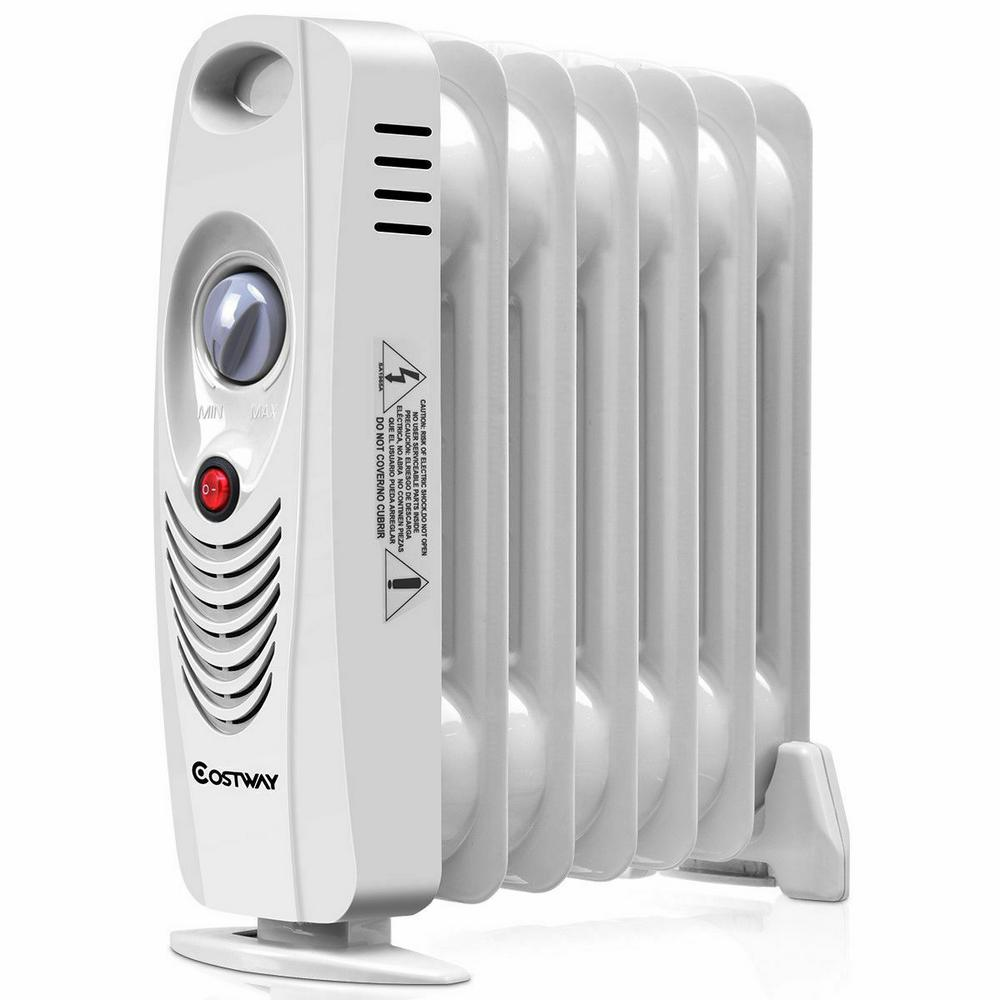# Why Electric Home Heat is So Expensive

1. Home
2. /
3. Electrical
4. /
5. Why Electric Home Heat...Heat is so expensive! Especially is this so for electric heat. Why?

Electricity flowing through a conductor obeys the simple mathematical relationship,

E = IR

That equation reads: electromotive force (E) equals current (I) times resistance (R). Since the power consumed (P) equals the current times the voltage,

P = EI = IR x I = I2R

P = I2R

This equation informs us that the power consumed by a device is equal to the square of the current (that is, the current times itself, I x I), times the resistance to current flow of the device.

If an electrical conductor is very good—for example, a thick copper wire—the power consumed is quite small. This is because the resistance to electrical flow is small. If current is measured in amperes (“amps” for short) and resistance is measured in ohms, the unit of power consumed is in watts.

For most household meters, the rate of use of electric power is measured as kilowatt-hours. The measure of power itself is in kilowatts. For example: if power use is a steady 1,250 kilowatt-hours for 12 hours, the power consumed is 1,250 x 12 = 15,000 kilowatts.

Let’s consider the consumption of power of a simple length of wire and compare it with the consumption by a 100-watt incandescent light bulb and, also, for a typical, portable, electric room-heater. Afterward, we’ll discuss why there is such a difference of electrical consumption for each of these three “devices.”

## Copper Wire

Typical electric home receptacles require 12-gauge (0.0808” diameter) copper wire. Its resistance is 0.001588 Ω (ohms) per foot. If the wire is 1000 feet in length, its total resistance is 1.588 Ω. This is very small indeed. If the current passing through the wire is at its maximum rating of 20 amps, the power consumed by the wire itself is

P = (20)(20)(1.588) = 635.2 watts = 0.6352 kilowatts

Generally, the wire will be required to consume less than this amount, since current demand will be less than the maximum.

## A 100-Watt Light Bulb

Clearly, one thousand feet of copper wire is a considerable amount of wire! In comparison, the approximately one inch filament of an incandescent bulb consumes a great deal of power. Just four light bulbs consumes the same amount of electricity as all that wire. When hot, the filament of a 100-Watt incandescent light bulb is approximately 144 Ω. Using the same power equation to solve for the current required to operate the bulb, we get

100 Watts = I2 (144 ohms)

I = √ 100/144

I = 0.833 amps

## Electric Home Heating

Now I use a heater—merely an auxiliary heater—in my bedroom during the winter months. It provides only a percentage of the heat required to warm my room. It is rated to use 12.5 amps, while providing 15,000 watts of heating power. This means my auxiliary electric home heating unit uses as much power as 150 one hundred-Watt light bulbs.

## Heat is So Expensive – Conclusion

The electric power consumed by a device depends upon its resistance, but even more importantly upon the current it uses. This is because the term involving the current is squared. It’s why electric home heat is so expensive!

Note: You might also enjoy Should Houses Switch to DC Power?

References:

### 2 thoughts on “Why Electric Home Heat is So Expensive”

• I had to read the first paragraph a couple of times to remind myself of those electricity equations I had to learn nearly 60 years ago. I take it your “electromotive force” is the “voltage”. I remember V=IR and Watts = Volts X Amps? I think that’s the same as you are saying above? Electricity certainly is expensive and it’s hard to do without it when the weather is cold.

• Quite so. Yes to both.